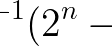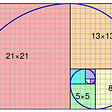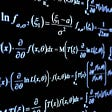# Part 3: Simple Matrices and Transformations

## New March 29th Presentation Date

In this part of my linear algebra series, I’ll be going over what a linear transformation is, and how it applies to matrices and matrix multiplication. I found this part of linear algebra to be very visual, a perspective of math that I’ve been trying to adopt recently. I had heard of transformations in the past, but that mainly pertained to altering the “parent functions” of parabolas so that their shapes changed. Linear transformations in the context of linear algebra are a little bit different: they actually alter the space, or plane, that vectors are placed on.

## Definition

For a linear transformation to be valid, a few conditions must be met.

• In a standard x-y plane, the x-axis and y-axis and all of its gridlines are straight. Linear transformations move these gridlines, but they can not end up being curved.
• The origin must remain at the same location.
• Gridlines remain parallel and evenly spaced from one another.
• As a result, vectors that get altered from the linear transformation don’t become curved either.

One common example of a linear transformation is a 90 degree rotation, either to the left or the right. The gridlines are clearly moved and remain parallel and evenly spaced, and the origin doesn’t magically shift to a point that isn’t (0,0). We can also imagine that if we take any vector and rotate it 90 degrees, it won’t become curved.

## Coordinate Basis

Before we dive into how matrices are applied, we must understand what the basis of a coordinate system is. In my words, this basis is like a default for how all vectors can be formed. In other words, each vector is created based on some alteration of the “basis vectors,” which are called “i-hat” and “j-hat.” To define these two vectors, “i-hat” represents the vector that has a magnitude of 1 moving directly to the right of the origin, an “j-hat” represents the vector that has a magnitude of 1 moving directly up from the origin. View below a visual depiction of the two.

As mentioned before, we can now think of any vector in space as the sum of some manipulation of i-hat and j-hat.

## Now On Towards Linear Transformations

We now have the fundamental knowledge to dive into linear transformations! The key to linear transformations is the tracking of the “basis vectors.” We know that in regular space, i-hat rests at (1,0) (in vector notation, this would be an upwards rectangle with the 1 at the top to represent x) and j-hat rests at (0, 1). Now, imagine that space gets shifted around, but under the conditions of our linear transformation. Now, the places that i-hat and j-hat land at will change. These new places are the key, and we can represent them in a simple 2x2 matrix.

In this example, we have the vectors where i-hat and j-hat end up falling at after undergoing a linear transformation. Take a second to visualize this in space. What is it like in comparison to the original resting spots of i-hat and j-hat? Now that we’ve visualized, we get to the fun part. Using this simple matrix, we can now find out where any vector in space would land at if it was applied the original linear transformation. To find out how to do this, we simply multiply the vector by the matrix.

Let’s look at an example.

In our example, what would happen to the vector (2,2) (again, pardon the notation)?

When multiplying the vector by the matrix, we can split the work such that the “x-component” of the vector gets multiplied by the component of the matrix that represents i-hat, and the “y-component” of the vector gets multiplied by the component of the matrix that represents j-hat; then, we add these two vectors together. Essentially, we’re just seeing what the transformed i-hat will do to the part of the vector that represents x-magnitude, and same with the transformed j-hat. We can add these two vectors together because any vector is represented by the sum of scaled versions of i-hat and j-hat. Look above for the sample calculation.

## Reflections

Once again, I’ve come to appreciate visual representation in mathematics. Throughout my journey learning about linear transformations, I found that visualizing the “basis vectors” moving around in space allowed me to comprehend what was going on. Right now, the math, number crunching, aspect of my introduction to linear algebra isn’t so difficult. But, understanding why it works gets my brain working more. Also, shoutout to 3Blue1Brown’s video series. It’s great, and it has awesome animations.

--

--

--

## More from Albert Ming

18 || High School Senior || Stats & Math

Love podcasts or audiobooks? Learn on the go with our new app.

## Part 1: Matrix Definitions## Monty Hall Problem will drive you crazy## Primes and The Powers of Two## Division By Zero In Computing — Does It Make Sense?## What is the Difference Between Speed and Velocity? — The Prodigious## Part 4: Determinants## The Brownian Bridge Process## Albert Ming

18 || High School Senior || Stats & Math

## Part 5: Revisiting Systems of Linear Equations## Is math invented or discovered?## Building your mathematical understanding: the “newcomer” approach## The World of Mathematics